# Sine Bar [Calculator, Sine Vise, Sine Plate, Easy Guide]

We give the Sine Bar, Sine Plate, and Sine Vise a special place in angle measurement because they’re used both for measuring and for setting when specific angles other than 90 degrees are important.  In fact, the sine bar is probably the primo metrology tool for working with angles.

Here’s a typical Sine Bar: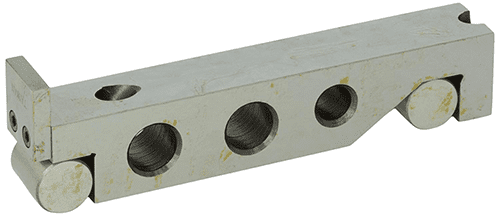Typical Sine Bar set from Fowler.  About \$145 on Amazon

There are cheaper versions to be had, but you want to make sure the one you get is precision ground and well made to ensure its accuracy.

The angle of a Sine Bar or Sine Plate is established using precision spacers under the rolls.  The rolls are the sine block two cylinders at either end.  They serve as pivots, and they’re cylindrical so there’s a line of contact between the surface it rests on and the Sine Bar or Sine Plate.  They should be of equal diameter.  The precision spacers used with sine bars are usually gauge blocks.

For comparison, here is a Sine Plate: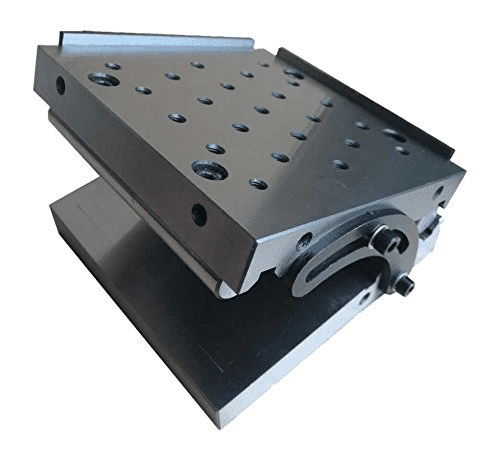Sine Plate.  About \$207 on Amazon

The Sine Bar is all about setting up an exact angle, while the Sine Plate is all about supporting a part or perhaps a piece of fixture at that angle.  A sine plate is also precision ground and uses the two cylinders.  Sine plates are much wider than sine bars.  A sine plate may also be called a sine table.

#### How to Use a Sine Bar

Here’s an example of Sine Bar usage where it is sitting on a slip gauge block stack: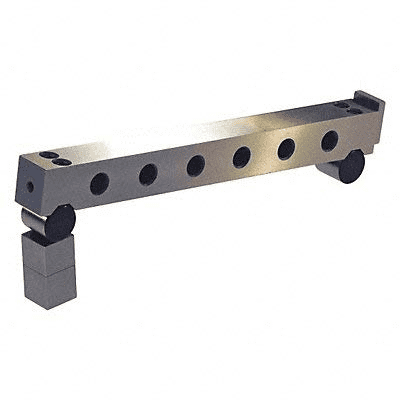Think of Sine Bars as converting a height to an angle, because that’s essentially what they do.  We will typically use a Gauge Block stack to establish the height and thus the angle.

Sine Vise

We can also get Sine Vises like this: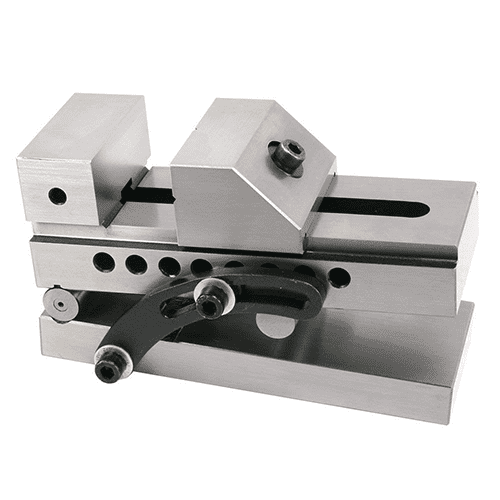Sine Vise.  About \$142 from Amazon

## Sine Bar Formula

The formula that relates the angle of the setup to the height of the gauge blocks is:

Sin( Angle ) = H / L, where H is the height and L is the length center to center (center distance), between the rolls.

Sine Bar Example

Let’s go through a sine bar formula example.  Suppose we want to set up a 30 degree angle.  So, let’s rearrange our formula a bit to give us the required sine bar elevation (H):

H = Sin( 30 degrees ) * L

The Sin of 30 degrees is 0.5.  So, we want the height to be half the length between the rolls.  For a 5″ long (center distance) Sine Bar, we would therefore need a gauge block height that is 2.500″ high.

Pretty easy, right?  And the formula works for sine plates and sine vises too.

But we can make it even easier with a special Calculator.

## Sine Bar Calculator

With this Calculator, you don’t need to remember formulas or track down  your calculator.  Instead you use a purpose-made piece of software that makes either finding the correct stack height for a given angle or figuring out what the angle is from a stack height easy.  In addition, it knows all about gauge blocks and will find the minimum number of standard sizes to make up any given height.

Our G-Wizard Calculator software has a built-in Sine Bar Calculator that looks like this: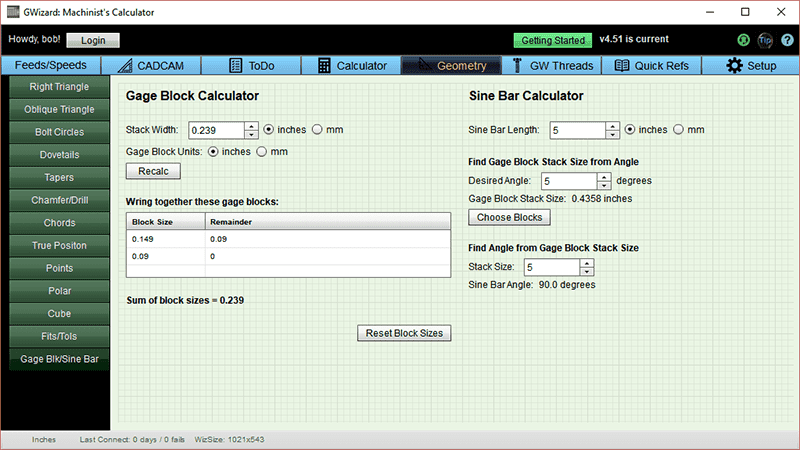You can get lifetime use of this handy calculator free, just for trying the free trial of G-Wizard.  In fact, you get free access to a whole slew of cool machinist’s geometry and math calculators.

Note how the Calculator is split into two components:

• Gauge Blocks Calculator
• Sine Bar Calculator

The two work together, but let’s focus on how to use them.

To calculate gauge block height for a particular angle:

1. Enter your sine bar length (aka “Center Distance”).  Common sizes in the US are 5 and 10 inches long.
2. Enter the desired angle.  For example, the screen shot shows 5 degrees.
3. The size for the Gage Block Stack will be shown.  The screen shot shows 0.4358.
4. Click the “Choose Blocks” button to see which blocks to stack to make that height.  Here’s the result after we clicked the button: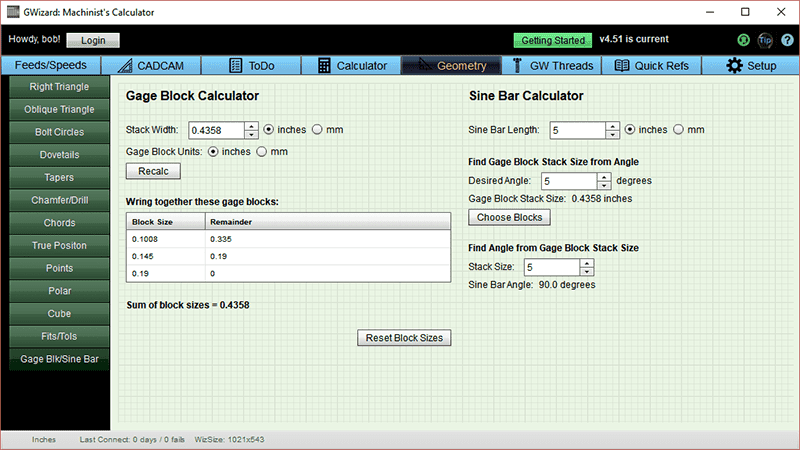You can see G-Wizard recommends 3 blocks for this angle.

Using a Sine Bar to Measure an Angle

There are a lot of ways to use a Sine Bar to measure an angle with great precision.  For example, you could create a setup like this: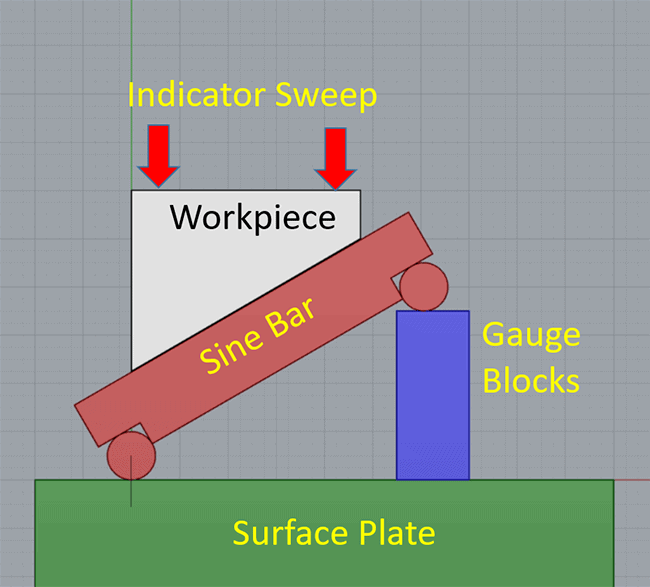How to measure angles using sine bars and gauge blocks on a surface plate.

Adjust the height of the Gauge Blocks until you can sweep an indicator across the top of the workpiece and the needle is steady, proving the surface is level.  Once you have that condition, you can use the Sine Bar Formula to determine the exact angle of the workpiece.  The surface plate ensures the overall accuracy of the setup.

Tip:  Use a Planer Gauge instead of a Gauge Block stack.  It’s faster and you can mic the Planer Gage to find the height.

Once you have the Stack Size measured, use G-Wizard Calculator to determine the angle: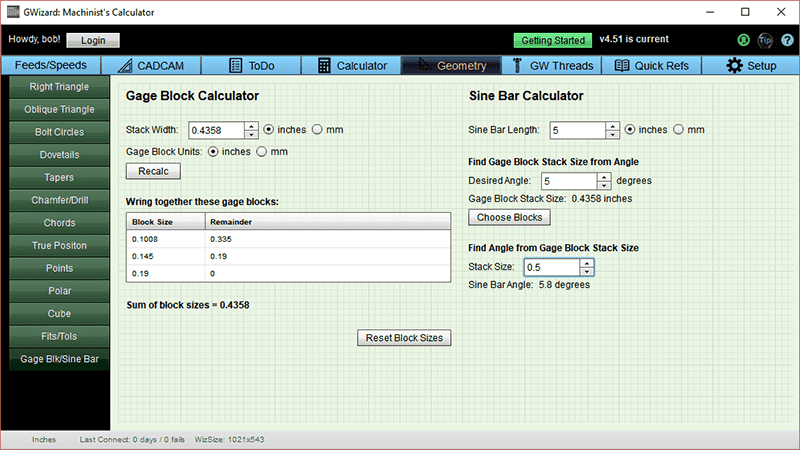As the screen shot shows, a stack size of 0.500″ means the angle is 5.8 degrees.

### Conclusion

Using these basic sine bar principles, you can either setup parts for machining at a desired angle or measure angles on parts with great precision.  As you can see, it’s not very hard to do, especially if you have a nice calculator like G-Wizard to handle the math.

While this is all fresh on your mind, go ahead and give it a try.  Measure some known angles using these techniques and see what you come up with.  Pretty soon it’ll be second nature!

And don’t forget to grab your free trial of G-Wizard.  The sine bar calculator will keep going for life, even after the trial expires.## Like what you read on CNCCookbook?

Join 100,000+ CNC'ers!  Get our latest blog posts delivered straight to your email inbox once a week for free. Plus, we’ll give you access to some great CNC reference materials including:

• Our Big List of over 200 CNC Tips and Techniques
• Our Free GCode Programming Basics Course
• And more!